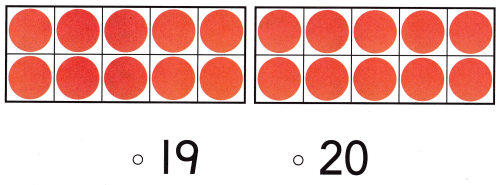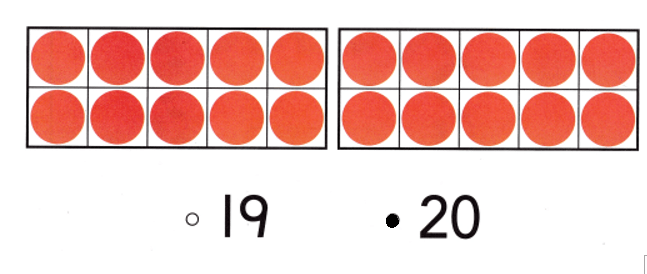# Texas Go Math Kindergarten Lesson 8.5 Answer Key Model, Count and Write 20

Refer to our Texas Go Math Kindergarten Answer Key Pdf to score good marks in the exams. Test yourself by practicing the problems from Texas Go Math Kindergarten Lesson 8.5 Answer Key Model, Count and Write 20.

## Texas Go Math Kindergarten Lesson 8.5 Answer Key Model, Count and Write 20

Explore

DIRECTIONS: Use counters to model 20. Draw the counters. Trace the number and word.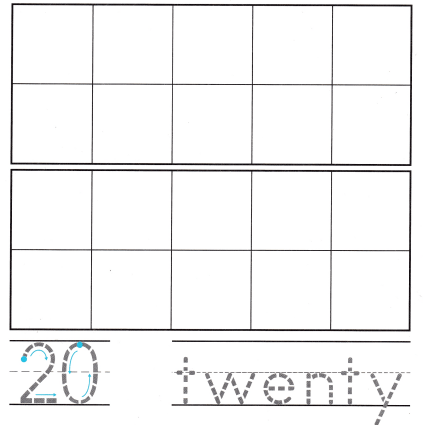Explanation:
Number of counters = 10 + 10 = 20 or Twenty.

Share and Show

DIRECTIONS: 1. Count and tell how many. Write the number. 2. Use cubes to model the number 20. Draw the cubes. 3. Use the cubes from Exercise 2 to model ten-cube trains. Draw the cube trains.

Question 1.Explanation:
Number of oranges = 10 + 10 = 20 or Twenty.

Question 2.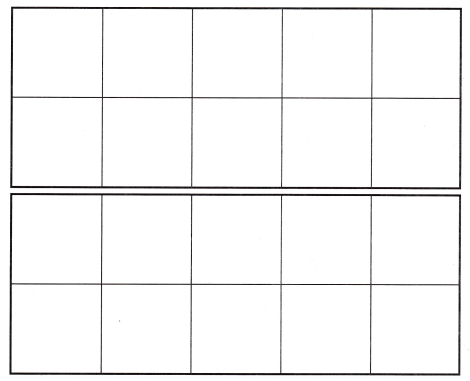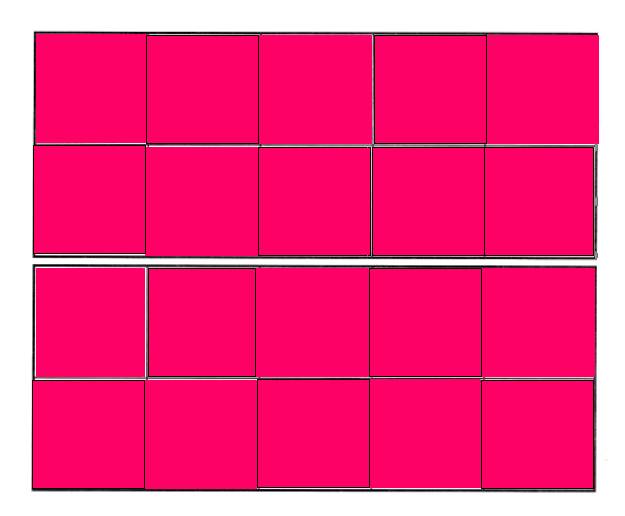Explanation:
Number of cubes = 10 + 10 = 20 or Twenty.

Question 3.Explanation:
Number of cubes = 10 + 10 = 20 or Twenty.

DIRECTIONS: 4-5. Count and tell how many pieces of fruit. Write the number. Tell a friend how you counted the fruit.

Question 4.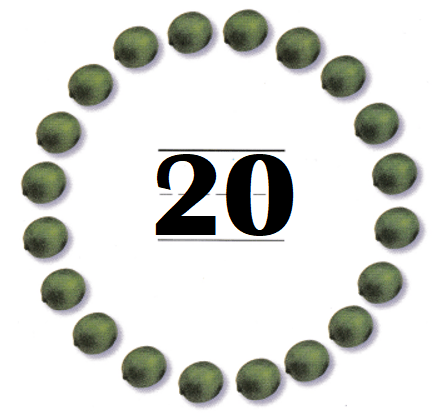Explanation:
Number of fruits = 10 + 10 = 20 or Twenty.

Question 5.Explanation:
Number of apples = 10 + 10 = 20 or Twenty.

HOME ACTIVITY • Draw two ten frames on a sheet of paper. Have your child show the number 20 by placing small objects, such as buttons or dried beans, in the ten frames.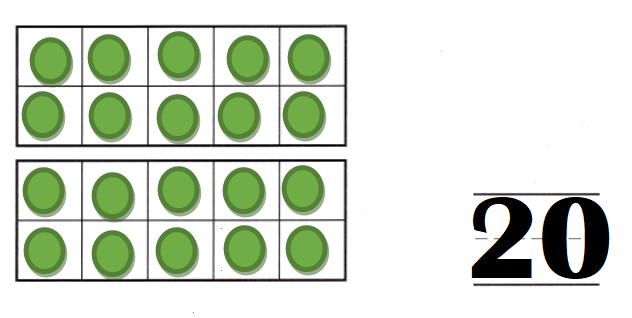Explanation:
My kid took buttons and placed them in the frames.
Number of buttons = 10 + 10 = 20 or Twenty.

DIRECTIONS: 6. Circle to show 20 beads. 7. How many of each color bead did you circle? Write the numbers. Tell a friend about the number of each color bead. 8. Choose the correct answer. What number does the model show?

Problem Solving
Question 6.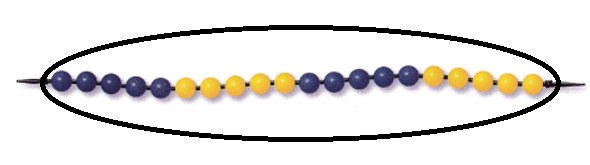Explanation:
Number of blue beads = 10.
Number of yellow beads = 10.
= 10 + 10
= 20 or Twenty.

Question 7.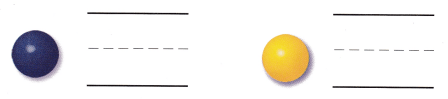Explanation:
Number of blue beads = 10.
Number of yellow beads = 10.
= 10 + 10
= 20 or Twenty.

Question 8.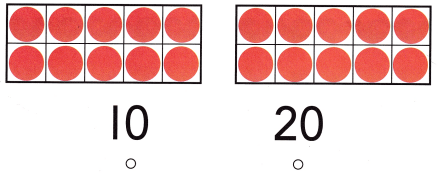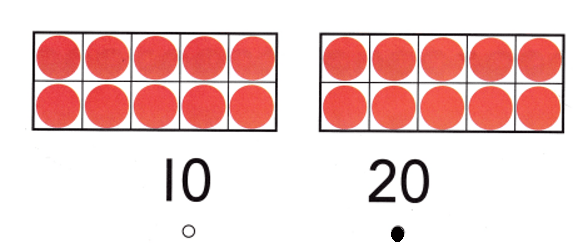Explanation:
Number of counters = 10 + 10 = 20 or Twenty.

### Texas Go Math Kindergarten Lesson 8.5 Homework and Practice Answer Key

DIRECTIONS: 1-2. Count and tell how many pieces of fruit. Write the number. Tell how you counted the fruit.

Question 1.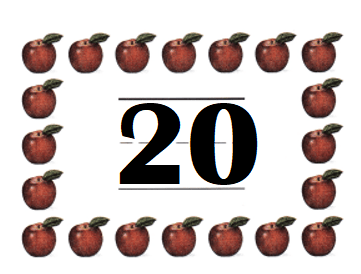Explanation:
Number of apples = 10 + 10 = 20 or Twenty.

Question 2.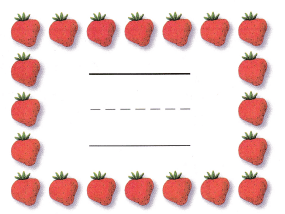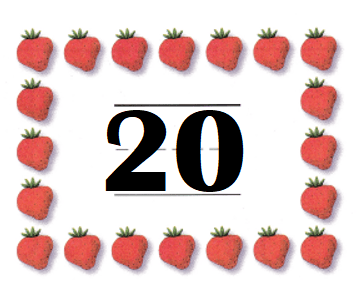Explanation:
Number of strawberries = 10 + 10 = 20 or Twenty.

DIRECTIONS: Choose the correct answer. 3-5. What number does the model show?

Lesson Check
Question 3.Explanation:
Number of counters = 10 + 10 = 20 or Twenty.

Question 4.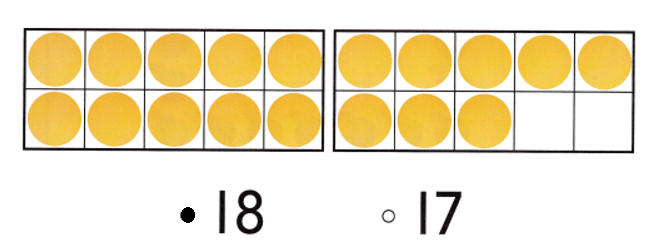Explanation:
Number of counters = 10 + 8 = 18 or Eighteen.

Question 5.